## 10/26/09

### Dramatic decline in labor force participation

Michael Mandel at “Economics Unbound” is really interested in labor force participation rate (LFPR). He devoted couple previous posts to related problems in an attempt to explain the evolution of labor force participation rate and productivity by some modern and fancy reasons. It is not worth to repeat his posts here and I just refer to Figure 1 as a general argument against any short-term force driving LFPR. The overall trajectory has a very clear picture of secular oscillations. Between 1965 and 2000, the LFPR was growing with just minor plateaus near 1980m and 1990. After 2000, the LFPR has been declining. This is a robust downward trend which hardly to be compensated by innovations, as Michael suggests.

In 2009, the LFPR has decreased from 66% to 65.4% in Q3 with average over the three quarters of 65.6%. A 0.6% drop in LFPR is a dramatic one. It corresponds to ~2,000,000 people leaving labor force in the US almost at once! (It is worth noting that such a drop may severely affect the rate of unemployment because people without job are more likely to leave labor force). According to our model , this the decline in the LFPR was expected in 2010. However, the population estimates, which are used for the prediction, have never been accurate enough for sharp timing. In any case, the model developed in [1-3], which links LFPR and productivity in developed countries to real GDP per capita has proved its consistency. The next two to three years should serve for further validation, as Figure 2 assumes.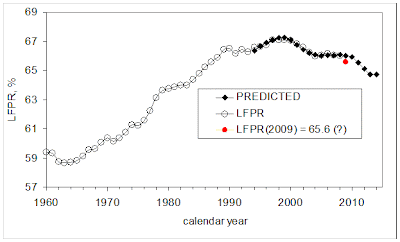Figure 1. The evolution of LFPR between 1960 and 2009.Figure 2. The observed LFPR and that predicted from real GDP per capita. We expect the LFPR to fall down to 64.5% by 2013.

The observed increase in productivity is directly related to the decrease in the LFPR. As a consequence, it was also well predicted by our model in . We used the projection of the number of 9-year-olds from the number of 1-year-olds for the prediction of real GDP per capita in the 2010s. Since 2010, the productivity has to be growing, as Figure 5 in , demonstrates.

a)b)Figure 5. Prediction of the number of 9-year-olds by extrapolation of population estimates for younger ages (1- and 6-year-olds).
a) Total population estimates. The time series for younger ages are shifted ahead by 8 and 3 years, respectively.
b) Change rate of the population estimates, which is proportional to the growth rate of real GDP per capita. Notice the difference in the change rate provided by 1-year-olds and 6-year-olds for the period between 2003 and 2010. This discrepancy is related to the age-dependent difference in population revisions.
A downward trend in productivity, as has been observed since 2003, will turn to an upward one in the 2010s. This also means an elevated growth rate of real GDP per capita during the period between 2010 and 2017.

References

 Kitov, I., Kitov, O., (2008). The Driving Force of Labor Force Participation in Developed Countries, Journal of Applied Economic Sciences, Spiru Haret University, Faculty of Financial Management and Accounting Craiova, vol. III(3(5)_Fall), pp. 203-222. http://www.jaes.reprograph.ro/articles/3_TheDrivingForceofLaborForceParticipationinDevelopedCountries.pdf

 Kitov, I., Kitov, O., (2008). The driving force of labor productivity, MPRA Paper 9069, University Library of Munich, Germany, http://ideas.repec.org/p/pra/mprapa/9069.html
http://mpra.ub.uni-muenchen.de/9069/01/MPRA_paper_9069.pdf

 Kitov, I., Kitov, O., (2009). Modelling and predicting labor force productivity, MPRA Paper 15152, University Library of Munich, Germany, http://mpra.ub.uni-muenchen.de/15152/01/MPRA_paper_15152.pdf

## 10/10/09

### Unemployment in Japan: now falling to the long-term level around 5%

In June and August 2009, we posted on the evolution of unemployment in Japan [P1] and [P2], which predicted the rate of 6.0% in August 2009. In the latter post we had to update the prediction and reduce the expected level of unemployment, as dictated by new readings of labor force. Moreover, we found that the level of unemployment in July was likely the peak value and the rate of unemployment in Japan would be decreasing since August 2009 according to the long term forecast (Kitov; 2006, 2007).
On the 2nd of October, the reading for August 2009 was reported by the Statistics Bureau of Japan. The (seasonally adjusted) rate for August was measured at 5.4%, i.e. 0.3% lower than in July. This observed decline is in line with that based on the model linking the rate of unemployment, UE(t), to the rate of change of labor force, dLF(t)/LF(t):

UE(t)= -1.5*dLF(t)/LF(t) +0.045 (1)

Figure 1 updates the observed and predicted curves for 2009. Because the accuracy of short-term estimates provided by labor surveys is not high, the monthly estimates of unemployment and labor force are prone to large measurement errors. The discrepancy between the observed and predicted curves likely manifests the problems with measurements. Figure 2 presents a mid-term view. In 2007 and 2008, the predicted unemployment was lower that the observed one, but in 2009 both variables are essentially the same (see Figure 1). In the long-run, the rate of unemployment in Japan will asymptotically approach 5.2% (Kitov, 2007), as displayed in Figure 3.

From relationship (1), we can conclude that the rate of unemployment in 2009 will likely undergo additional decline. The peak rate of unemployment is left behind, but its further decrease will accompany the decline in the level of labor force.

References
Kitov, I., (2006). The Japanese economy, MPRA Paper 2737, University Library of Munich, Germany, http://ideas.repec.org/p/pra/mprapa/2737.html
Kitov, I., (2007). Exact prediction of inflation and unemployment in Japan, MPRA Paper 5464, University Library of Munich, Germany, http://ideas.repec.org/p/pra/mprapa/5464.html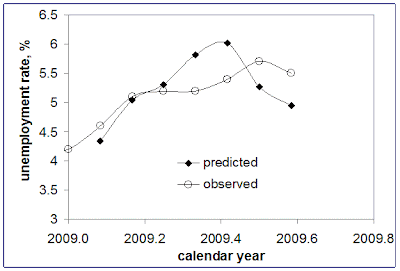Figure 1. Observed and predicted rate of unemployment in Japan in 2009.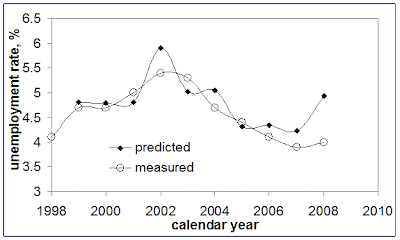Figure 2. Observed and predicted rate of unemployment in Japan between 1998 and 2008.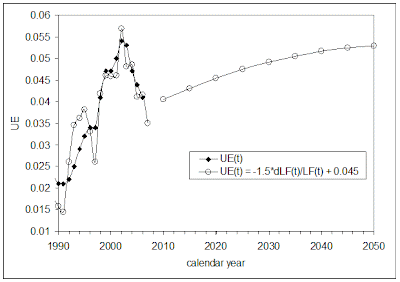Figure 3. Prediction of the evolution of unemployment rate in Japan between 1990 and 2050 (Kitov, 2007).
Notice excellent prediction between 1998 and 2007 with the peak value in 2001.

## 10/9/09

### Semi-annual report on SP 500: from April to September 2009

This is a semi-annual report on the evolution of S&P 500 index. It compares the actually observed monthly closing levels to those predicted by our model, as originally presented in . The major conclusion is that the prediction of a monotonic growth in S&P level made in March 2009 is correct. There is no indication that the growth will end before May 2010, where we foreseen the turn to a negative slope. Accordingly, the S&P 500 annual return will soon reach positive figures and will be growing at an accelerated rate till May 2010.

The model links S&P 500 annual returns to the number of 9-year-olds and has passed econometric tests for cointegration, with goodness-of-fit reaching 0.9. Since the number of 9-year-olds can be accurately predicted using younger cohorts, one can predict S&P returns at a several-year horizon. (Standard demographic projections may be used to predict over a decade ahead.)

We revealed the link between S&P 500 and the number of 9-year-olds in December 2007 using historical data since 1985. Since the very beginning of 2008, we have been carefully tracking the evolution of S&P 500. The original model actually predicted a sharp fall in 2008 . (As in many scientific studies, the attempt to improve the original model, in order to fit data between 2003 and 2007, had failed, as we reported in this blog at several occasions.) The re-calibrated version of the original model developed in March 2009 is as follows:

Rp(t) = 165dln[N3(t+6)] - 0.17 (1),

In (1), Rp is the 12-month cumulative return; N3 is the number of three-year-olds; t+6 – time shifted by six years ahead to extrapolate the number of 3-year-olds into the number of 9-year-olds; dln is the rate of growth, i.e. the monthly increment in N3 normalized to the contemporary level. The time step is one month or 1/12 of a year.
Figure 1 compares the initial prediction of the S&P 500 evolution, the observed trajectory for the period since February 2009, and a new prediction till 2011. Relationship (1) is used with the number of 9-year-olds extrapolated from the number of 3-year-olds. In March 2009, we used a preliminary calibration of the original model and assumed the monthly increment in S&P 500 would be 80 points. This assumption was too optimistic and the growth was weaker. The original prediction is shown by blue lin with solid circles in Figure 1, and the observed values are shown by red diamonds. The black line represents an updated prediction since September 2009. It reproduces the old prediction but with a 46-point monthly increment since October 2009, as discussed below.

All in all, Figure 1 presents strong evidence in favour of our original model. Six months of almost monotonic growth were not expected by many market players in March 2009. The next nine months should bring additional validation to the model. The most important event will be the turn in May 2010. But the growth before this date is of crucial importance as well.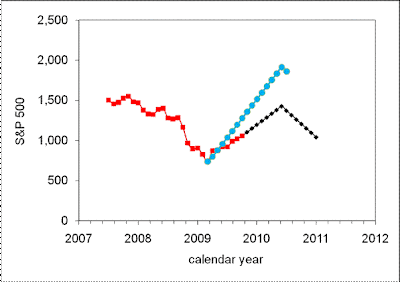Figure 1. The evolution of S&P 500. Blue line - the original prediction using (1) with 80 unit per month increment. Red line – observations. Black line – the updated prediction since October 2009 with a 46-point monthly increment.

In any case, the model predicts a sudden drop in 2008 and 2009 to the level of 700, which has been followed by constant growth to the level 1050 in September 20009. Initially, we estimated the peak value in 2010 as 1800. But the last six months demonstrated the necessity to re-calibrate the model using new data. (In physics, even fundamental constants are under permanent re-estimation, and empirical and even fundamental models are constantly recalibrated.) Therefore, we also re-estimated coefficients in (1) to fit the last six S&P 500 (monthly) readings. The new model is as follows:

Rp(t) = 135dln[N3(t+6)] - 0.17 (2),

Actually, we needed to reduce the coefficient of linear term from 165 to 135 with free term unchanged. Figure 2 depicts the updated prediction of the S&P 500 annual returns. The peak S&P 500 value in May 2010 should be 1425 (not 1800) if the future increment will be 46 points per month, as observed between March and September 2009.Figure 2. Observed and predicted S&P 500 returns. The September level of S&P 500 index is 1057. The past six months are relatively well predicted.

Conclusion
Between 1985 and 2009, the S&P 500 returns can be accurately described by population estimates. The model based on the number of 9-year-olds produces a time series which is cointegrated with the S&P 500 returns, i.e. reveals a weak causality, as proved by the cointegration tests.

The re-calibrated model predicts the continuation of S&P 500 growth into 2010 with the peak level of ~1400 in May. The annual returns will reach positive zone soon and also peak in May 2010 at the level of 50% to 70%.

References
 Kitov, I., Kitov, O., (2007). Exact prediction of S&P 500 returns, MPRA Paper 6056, University Library of Munich, Germany, http://ideas.repec.org/p/pra/mprapa/6056.html

## 10/6/09

A deflationary period is coming. As we wrote in 2006, deflation should start in 2012. Some funds (Pimco, for example, as Bloomberg reports) are getting prepared to these poor times. Do not miss the quick start and re-visit our old paper for the prediction of the deflationary period lenght:

Inflation, unemployment, labor force change in the USA
Ivan O. Kitov
Abstrac
t
A model is developed linking the measured inflation (consumer price index or GDP deflator), unemployment and change in labor force. During the last twenty-five years, unemployment in the USA has been a lagged linear function of inflation. In turn, inflation has also been a lagged linear function of relative change in labor force with time. The lag is currently three years. Only a small decrease in labor force participation rate is currently observed in contrast to a strong increase between 1965 and 1990. According to the indicated relationship, the well-known stagflation period clearly resulted from the lag: the sharp increase in inflation coincided in time with the high unemployment induced by the high inflation period two years before. One can predict the unemployment rate in the USA in the following two years within the accuracy of inflation measurements. For example, the end of 2005 is a pivot point from a period of decreasing unemployment to one of moderate growth from 5% in 2005 to 6% in the middle of 2008. Starting in 1960, cumulative values of the observed and the model predicted unemployment are in agreement with the lag between inflation and unemployment. Inflation is defined by a lagged linear function of rate of change in labor force. The observed and predicted inflation almost coincide for the last forty years of annual measurement values, smoothed by a five-year wide moving window curves and as cumulative curves as well. Deviation of the curves before 1960 can be explained by a degraded accuracy of the measurements. A severe decrease in the rate of change of labor force is expected after 2010. This drop can potentially induce a long-term deflationary period. The same effect has been observed for Japan starting in 1990. There are numerous implications of the results for monetary and social policy-makers. The most important is an absence of any means to control inflation and economic growth except though a reasonable labor policy. In addition, some urgent measures are necessary to prevent the start of a deflationary period in 2010-2012.

## 9/29/09

### CPI components in Japan: Medical care and education

As we discussed many times in this blog, Japan has an eternal problem with deflation. The headline CPI and its components are on a long-term decline. It will continue another 40 years because the level of labor force in Japan has been and will be decreasing in line with the ageing and overall depopulation. Today, Japan Statistics released an estimate of the consumer price index for August 2009 - http://www.stat.go.jp/english/data/cpi/1581.htm.

It is instructive, that the only two components (from ten), which did not suffer annual decrease, are the medical care and education. This is in agreement with the extraordinary high rate of growth in both these components in the USA .
Despite the surging prices for medcare and education, the US is awaiting the fate of Japan - a longer deflationary period since 2012.

## 9/25/09

### Goldman Sachs on oil in 2009 and 2010

Reuters cites an updated forecast on oil demand and price just issued by GS: \$85 per barrel by the end of 2009. Currently, oil price is down to \$66-67, but it should reach \$100 in 2009.

## 9/24/09

### The Feds must bail out Lehman Brothers

There is a fundamental question on relative merits of the previous bank bailouts - which bank did deserve a bailout and how much would it really cost? Our model of stock price, developed in articles and posts, provides an additional measure of the size of bank problems - total debt. One can estimate the debt as a product of the number of shares and their market price, which was negative for the bailed out and not bailed out banks. We base our estimate on relevant stock prices described in this blog. Table 1 lists the number of stocks, their peak negative price, and the debt:

Table 1.
....................vol............ price,\$.......debt,\$
LEH...... 689,000,000.......... -40...... -2.76E+10
C ..........11,000,000,000..... -10.......-1.10E+11
AIG....... 134,000,000........ -870 ......-1.17E+11
FRE...... 648,000,000......... -40 ........-2.59E+10
FNM...... 1,110,000,000...... -45 .......-5.00E+10
(see fictitious stock price estimates for Citigroup and AIG in Figures below)

The table shows that Lehman Brothers had smaller problems than Citigroup and AIG. So, it was was easier to bail out LEH from purely mathematical and financial point of view.

Also, the joint negative price of AIG, FRE and FNM is less than 200bn. It could be some (deliberate?) miscalculation of the >1000bn help package. The toxic debt might be bigger than the total debt associated with stock prices, but there should be unaccounted positive assets.## 9/23/09

### Beyond the bankruptcy – Fannie Mae, Freddie Mac, and Lehman Brothers

Introduction
In several previous posts on bankruptcy, we have described the evolution of share prices for banks just before their bankruptcy or on their approach to negative prices: Colonial Bank (CNB), the CIT Group (CIT), American International Group (AIG), and Lehman Brothers (LEH). It was shown that the CNB sank below the edge of bankruptcy and CIT is on the brink with unclear future. AIG has been fluctuating around the waterline for a while, and LEH was definitely ready for a bankruptcy.

On the other hand, the CIT Group and AIG are bailed out, and thus, our model predicting share prices should fail to predict the artificially supported prices. Therefore, it would be instructive to estimate the fictitious evolution of share prices after the bankruptcies or alternative evolution after the bailouts. In this post we compare Lehman Brothers, Fannie Mae (FNM) and Freddie Mac (FRE). All three had a sharp fall in the second half of 2008.

Model and data
To predict share prices we use the 3-C model [1-3]: a share price, sp(t), is described with the following empirical function:

sp(t) = A*CPI1(t+t1) + B*CPI2(t+t2) + C*(t-2000) + D (1)

, where CPI1 and CPI2 are empirically determined CPI components; t is the calendar time; A, B, C, and D are empirical coefficients. Because the prices for goods and services (in the headline CPI) can lag or lead relevant share prices the time lags t1 and t2 are introduced. Both time lags can be positive or negative. In our model, we test all possible lags between 0 and +13 months. So, we chose the best from 34*33*14*14 ~ 200,000 possible models, with two extra degrees of freedom introduced by linear and free terms.

The full set includes 34 CPI components, which are tested as predictors for each share price. Among these components are major expenditure categories: the headline CPI (C), the core CPI, i.e. the headline CPI less food and energy (CC), food and beverages (F), housing (H), apparel (A), transportation (T), medical care (M), recreation (R), education (ED), communication (CO), and other goods and services (O). All components used in the model are not seasonally adjusted ones and retrieved from the Bureau of Labor Statistics.

Results
When applied to the LEH time series between July 2003 and September 2008, the 3-C model (1) gives the following empirical relationship:

LEH(t) = -10.40*FB(t-4) + 5.84*DUR (t+2) + 67.96*(t-2000) + 952; STDEV=\$4.73 (2)

, where t is the calendar time, FB(t) is the (not seasonally adjusted consumer price) index for food leading the LEH by four months, and DUR(t+2) in the index of durable goods lagging two months behind the LEH. Figure 1 compares the stock price predicted according (2) with the observed one. This model differs from that in the previous post in two aspects. First, it uses DUR instead of the personal care index (PC). This is not a fundamental change, however. Each empirical model based on two components of the CPI includes one index, which is sensitive to the change in a given share price, and one index, which expresses the overall reaction of prices for goods and services induced by the change in the share price.
Here I would like to illustrate the process on the example of isostatic compensation of ice floating in a water-filled tank. If you put some load on a piece of ice it will sink a bit deeper, but the level of water in the tank will also increase. Similarly, if some stock price increases it will affect prices of many goods and services, not only those related to the stock. There should be a component of the CPI which expresses the overall reaction the best. One can consider it as a reference component.

Then the difference between these two components maps the change in the price. Since there are many components of the CPI, which might represent the overall reaction of the CPI on the given price change, their exchange does not influence the model much. In the case of LEH, the defining component is the index for food (or food and beverages), and the reference component is the PC or DUR.

The empirical models for FNM and FER are as follows:

FNM(t) = -7.38*FB(t-8) – 8.47*R (t+3) + 42.57*(t-2000) + 2119; STDEV=\$4.42 (3)
FRE(t) = -6.07*FB(t-4) - 3.42*O (t-9) + 57.96*(t-2000) + 1917.8; STDEV=\$4.16 (4)

, where R is the index of recreation and O is the index for other goods and services in the CPI. Figures 2 and 3 compare the observed and predicted prices for FNM and FER, respectively.
All three empirical models just describe the evolution of corresponding prices using available CPI estimates. The models do not foresee stock the price evolution beyond current knowledge. However, since relevant CPI estimates are available for the last year, i.e. 11 months after the bankruptcy of Lehman Brothers, one can formally calculate the fictitious evolution of these share prices, as shown in Figures 1through 3. The LEH price demonstrates sustainable decrease between October 2008 and March 2009. Then the price regains some strength and approaches the zero line in August 2009. If bailed out in September 2008, the Lehman Brothers would start to grow in September 2009. One cannot say the same thing about AIG – it is still unstable.
The price of Fannie Mae lags behind both defining CPI components. Therefore, the prediction is possible only till April 2009. Unfortunately, the price continues its fall. There is no sign a turn. On the contrary, the price of Freddie Mac is well predictable into 2010. In March 2009, the price reached the bottom and then started to grow. It is still negative and will stay below the zero line in 2009.

Conclusion
We have predicted fictitious share prices of three companies. This prediction might be helpful for the assessment of future policy on Freddie Mac and Fannie Mae.Figure 1. Comparison of the observed and predicted LHE’s share price. The latter is obtained using (2).Figure 2. Comparison of the observed and predicted FNM’s share price. The latter is obtained using (3).Figure 3. Comparison of the observed and predicted FRE’s share price. The latter is obtained using (4).

References
 Kitov, I., Kitov, O., (2009). Predicting share price of energy companies: June-September 2009, MPRA Paper 15863, University Library of Munich, Germany, http://mpra.ub.uni-muenchen.de/15863/01/MPRA_paper_15863.pdf
 Kitov, I., Kitov, O., (2009). Modelling selected S&P 500 share prices, MPRA Paper 15862, University Library of Munich, Germany, http://mpra.ub.uni-muenchen.de/15862/01/MPRA_paper_15862.pdf
 Kitov, I., Kitov, O., (2008). Long-Term Linear Trends In Consumer Price Indices, Journal of Applied Economic Sciences, Spiru Haret University, Faculty of Financial Management and Accounting Craiova, vol. 3(2(4)_Summ), pp. 101-112.

## 9/22/09

### Predicting bankruptcy : the case of Lehman Brothers

We continue testing our model againts bancruptcy cases. Lehman Brothers presents an old but crucial case. Before we start our quantitative analysis, we have to clarify the the term "predicting" has a narrow sense of quantitative description. In other words, we do not forecast the bankruptsy, but decompose relevant stock price, sp(t), into a sum of two CPI components, a linear and a free term:

sp(t) = A*CPI1(t+t1) + B*CPI2(t+t2) + C*(t-2000) + D (1)

where CPI1 and CPI2 are defining CPI components; t is the calendar time; A, B, C, and D are empirically determined coefficients. Because prices for good and services can lag or lead relevant share prices the time lags t1 and t2 are introduced. Both time lags can be positive or negative. In our model, we test all possible lags between 0 and +13 months. So, we do not pretend that one could see the fall of LEH months before it had happened.

So, the empirical model describing the time history of LEH share price is as follows:

LEH(t) = -9.75*F(t-4) – 8.13*PC (t-2) + 94.9*(t-2000) + 2802 (2)

, where t is the calendar time, F(t) is the (not seasonally adjusted consumer price) index for food and beverages, leading the LEH(t) by four months, and PC(t-13) in the index of personal care leading the LEH(t) by 2 months. Or this model we used LEH prices between July 2003 and September 2008; the CPI components were taken till December 2008, i.e. three months ahead of the last LEH reading. Figure 1 demonstrates that the model predicted the fall below zero but three months later. Standard deviation of the residual is \$4.7.
Once again, this model could not be obtained in August 2008.Figure 1. Observed and predicted LEH share price.

### Predicting bank bankruptcy – the case of AIG

Update 1. There is a post at MarketBeat devoted to AIG : AIG Shares: Still Not Worth Anything. In general it supports our model with a negative estimate of the share price. The case is getting more and more exiting ...
In the previous post on bankruptcy, we have described the evolution of share prices for the Colonial Bank (CNB) and the CIT Group (CIT). It was shown that CNB sank below the edge of bankruptcy. (All share prices are retrieved from YahooFinance.) Here we investigate the case of AIG. It has been bailed out. Not considering political, economic and financial aftermaths of such a bankruptcy we focus on the prediction by our 3-C model: a share price, sp(t), is described with the following empirical function:

sp(t) = A*CPI1(t+t1) + B*CPI2(t+t2) + C*(t-2000) + D (1)

where CPI1 and CPI2 are defining CPI components; t is the calendar time; A, B, C, and D are empirically determined coefficients. Because prices for good and services can lag or lead relevant share prices the time lags t1 and t2 are introduced. Both time lags can be positive or negative. In our model, we test all possible lags between 0 and +13 months. So, we chose the best from 34*33*14*14 ~ 200,000 possible models, with two extra degrees of freedom introduced by linear and free terms.

The full set includes 34 CPI components, which are tested as predictors for each share price. Among these components are major expenditure categories: the headline CPI (C), the core CPI, i.e. the headline CPI less food and energy (CC), food and beverages (F), housing (H), apparel (A), transportation (T), medical care (M), recreation (R), education (ED), communication (CO), and other goods and services (O). All components used in the model are not seasonally adjusted ones and retrieved from the Bureau of Labor Statistics.
When applied to AIG time series between July 2003 and August 2009, the 3-C model (1) for AIG is as follows:

AIG(t) = -89.29*F(t) – 08.31*FB (t-13) + 1031*(t-2000) + 32666 (2)

, where t is the calendar time, F(t) is the (not seasonally adjusted consumer price) index for food and beverages and FB(t-13) in the index of food without beverages leading AIG(t) by 13 months.
Figure 1 compares the stock price predicted according (2) with the observed one. There are two dives of the predicted price below zero – March and August 2009, the latter being of -\$123. With standard deviation of \$102, the predicted price in August likely hints at unresolved problems with AIG. However, the negative price might also result from the uncertainty in F and FB measurements.
We will continue tracking AIG stock price and reporting our predictions.Figure 1. Comparison of the observed and predicted AIG’s share price. The latter is obtained using (2).

### Share price of select energy companies. Part 2Figure 12. Observed and 3-C predicted Halliburton share prices: HAL(t) = -3.66*EC(t-6) +2.42*CF(t+1) - 0.41*(t-2000) - 37, where EC is the index of education and communication and CF is the headline CPI less food. Standard deviation is \$2.35.Figure 13. Observed and 3-C predicted Hess CP share prices: HES(t) = 0.61*E(t+1) + 4.1*RENT(t-7) – 26.4*(t-2000) - 865.7, where EC is the index of education and communication and CF is the headline CPI less food. Standard deviation is \$5.92.Figure 14. Observed and 3-C predicted Massey Energy CP share prices: MEE(t) = 3.25*CSH(t+1) - 6.37*CFSHE(t-11) + 2.33*(t-2000) + 592.9, where CSH is the headline CPI less shelter and CFSHE is the headline CPI less food, shelter and energy. Standard deviation is \$6.30.Figure 15. Observed and 3-C predicted Murphy Oil CP share prices: MUR(t) = 0.83*T(t+1) – 6.25*HFO(t) + 6.20*(t-2000) + 655.9, where T is the index of transportation and HFO is the index of household furnishing and operations. Standard deviation is \$4.27.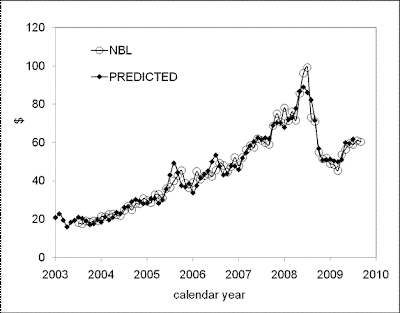Figure 16. Observed and 3-C predicted Noble Energy share prices: NBL(t) = 0.86*T(t+2) + 2.88*RENT(t-3) - 15.8*(t-2000) - 694.4, where T is the index of transportation and RENT is the index of rent. Standard deviation is \$3.97.Figure 17. Observed and 3-C predicted National Oilwell Varco share prices: NOV(t) = 4.13*C(t+1) – 10.87*HFO(t+1) - 9.26*(t-2000) + 641.5, where C is the headline CPI and HFO is the index of household furnishing and operations. Standard deviation is \$5.28.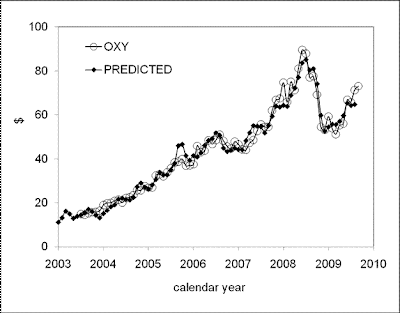Figure 18. Observed and 3-C predicted Occidental Petroleum share prices: OXY(t) = 0.73*T(t+1) + 1.17*MCS(t-5) - 12.36*(t-2000) - 405.5, where T is the index of transportation and MCS is the index of medical care services. Standard deviation is \$3.25.Figure 19. Observed and 3-C predicted Pioneer Natural Resources share prices: PXD(t) = - 3.24*EC(t-6) + 0.43*E(t+1) + 4.08*(t-2000) + 305.6, where EC is the index of education and communication and E is the index of energy. Standard deviation is \$3.73.Figure 20. Observed and 3-C predicted Rowan Companies share prices: RDC(t) = - 1.74*F(t-5) + 0.49*TPR(t+1) + 9.59*(t-2000) + 222.5, where F is the index of food and beverages and TPR is the index of private transportation. Standard deviation is \$2.85.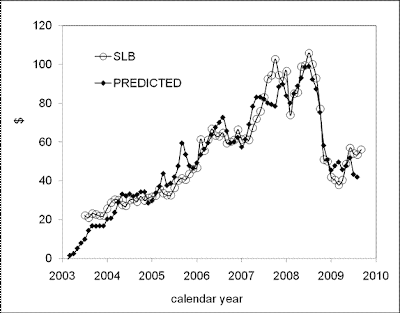Figure 21. Observed and 3-C predicted Schlumberger share prices: SLB(t) = - 5.47*F(t-11) + 0.93*T(t+1) + 36.9*(t-2000) + 695.9, where F is the index of food and beverages and T is the index of transportation. Standard deviation is \$7.24.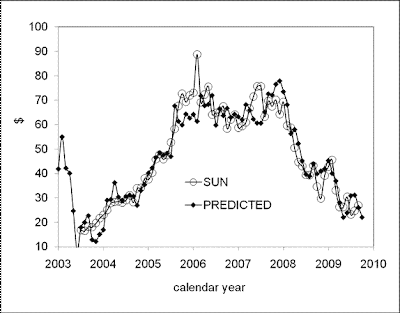Figure 22. Observed and 3-C predicted Sunoco share prices: SUN(t) = - 15.9*CO(t-8) + 10.17*HFO(t-8) - 19.8*(t-2000) + 257.7, where CO is the index of communication and HFO is the index of household furnishing and operations. Standard deviation is \$6.12.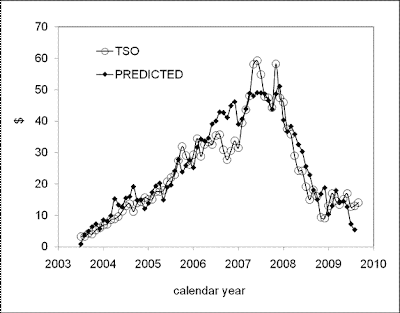Figure 23. Observed and 3-C predicted Tesoro share prices: TSO(t) = -2.85*F(t+2) - 5.44*FB(t-11) + 54.4*(t-2000) + 1273, where F is the index of food and beverages and FB is the index of food only. Standard deviation is \$6.12.Figure 24. Observed and 3-C predicted XTO Energy share prices: XTO(t) = 1.49*CSH(t+2) – 1.73*FB(t-6) + 9.87*(t-2000) + 18.1, where CSH is the headline CPI less shelter and FB is the index of food only. Standard deviation is \$3.29.

## 9/21/09

### Share price of select energy companies

Since September 16, 2009 the readings of the headline CPI and its components for August 2009 are available (we retrieve all CPI data from http://www.bls.gov/data). We recalculate our model for selected stock prices from the S&P 500 list. First was ConocoPhillips since it provides a good example of a company, which share price has been leading defining components of the CPI. Here we present several companied from the “energy” subcategory of S&P 500. It is instructive that these companies are rather different than similar in the evolution of share price and thus in defining CPI components.

In the previous posts and articles [1,2] we introduced several models with varying number of CPI components. In our view, the best one, we call it 3-C model, is seeking those two CPI components from 34 pre-selected ones, which minimize the difference between observed (monthly closing price adjusted for dividends and splits) and predicted prices for the period between July 2003 and August 2009. This model also includes free term (constant) and linear time term [3-6], which compensates well know linear (time) trends between various CPI components.

In the previous post we reported the empirical 3-C model for COP:

COP(t)= 3.41CF(t+1) - 7.17EC(t-6) + 7.22*(t-2000) + 145.76

where t is the calendar time, CF is the headline CPI less food, which lags behind COP by one months, and EC is the index for education and communication leading COP by six months. Standard deviation between the curves is \$3.96 for the period between July 2003 and August 2009. Figure 1 displays the observed and predicted prices.Figure 1. Observed and 3-C predicted COP’s share price.

Below we present analytic models and figures for several energy companies without comments. One can judge the accuracy of the predictions and the similarity/difference between the companies.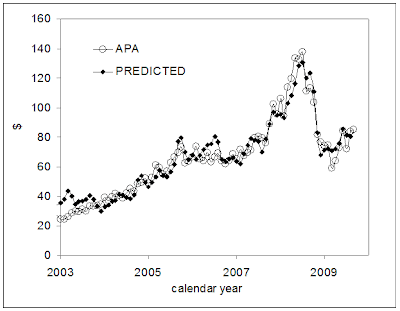Figure 2. Observed and 3-C predicted Apache Corp.’s share price: APA(t) = 1.35*TPR(t+1) + 3.21*MCS(t-7) + 47.20*(t-2000) - 973, where TPR is the index of private transportation, MCS is the index of medical care services. Standard deviation is \$6.36.Figure 3. Observed and 3-C predicted Anadarko Petroleum Corp.’s share price: APC(t) = 3.31*EC(t+2) – 1.74*CSHF(t-5) + 5.49*(t-2000) + 58, where EC is the index of education and transportation, CSHF is the headline CPI less shelter and food. Standard deviation is \$3.22.Figure 4. Observed and 3-C predicted Baker Hughes’ share price: BHI(t) = 4.93*F(t-5) – 0.79*T(t+1) + 32.53*(t-2000) + 662, where F is the index of food and beverages, T is the index of transportation. Standard deviation is \$5.30.Figure 5. Observed and 3-C predicted Peabody Energy Corp.’s share price: BTU(t) = 0.64*FU(t-4) + 1.36*TPR(t+1) – 8.37*(t-2000) - 266, where FU is the index of (housing) fuels and utilities, TPR is the index of private transportation. Standard deviation is \$4.35.Figure 6. Observed and 3-C predicted Cameron Intl. CP share prices CAM(t) = 0.64*TPR(t+1) + 2.65*RENT(t-4) - 17.1*(t-2000) - 610, where FU is the index of (housing) fuels and utilities, TPR is the index of private transportation. Standard deviation is \$3.38.Figure 7. Observed and 3-C predicted Chesapeake Energy Corporation share price: CHK(t) = 0.41*E(t+1) - 1.50*ED(t-7) - 12.1*(t-2000) + 102.7, where E is the index of energy and ED is the index of education. Standard deviation is \$2.70.Figure 8. Observed and 3-C predicted Consol Energy share price: CNX(t) = 6.11*C(t+2) – 2.62*ED(t-5) – 2.81*(t-2000) - 767.5, where C is the headline CPI and ED is the index of education. Standard deviation is \$5.65.Figure 9. Observed and 3-C predicted Chevron share price: CVX(t) = 0.25*E(t+1) + 2.76*MCS(t+4) – 37.6*(t-2000) - 716.1, where E is the index of energy and MCS is the index of medical care services. Standard deviation is \$3.26.Figure 10. Observed and 3-C predicted Devon Energy Corp.’s share price: DVN(t) = 0.79*T(t-3) + 0.46*E(t+2) – 0.68*(t-2000) - 162.7, where E is the index of energy and T is the index of transportation. Standard deviation is \$5.67.Figure 11. Observed and 3-C predicted Ensco share prices: ESV(t) = 2.23*CSH(t+1) - 2.61*FB(t-5) + 8.89*(t-2000) + 65.9, where CSH is the headline CPI less shelter and FB is the index of food only. Standard deviation is \$3.37.

References
 Kitov, I., Kitov, O., (2009). Predicting share price of energy companies: June-September 2009, MPRA Paper 15863, University Library of Munich, Germany, http://mpra.ub.uni-muenchen.de/15863/01/MPRA_paper_15863.pdf
 Kitov, I., Kitov, O., (2009). Modelling selected S&P 500 share prices, MPRA Paper 15862, University Library of Munich, Germany, http://mpra.ub.uni-muenchen.de/15862/01/MPRA_paper_15862.pdf
 Kitov, I., Kitov, O., (2008). Long-Term Linear Trends In Consumer Price Indices, Journal of Applied Economic Sciences, Spiru Haret University, Faculty of Financial Management and Accounting Craiova, vol. 3(2(4)_Summ), pp. 101-112.
 Kitov, I., (2009). Apples and oranges: relative growth rate of consumer price indices, MPRA Paper 13587, University Library of Munich, Germany, http://mpra.ub.uni-muenchen.de/13587/01/MPRA_paper_13587.pdf
 Kitov, I., Kitov, O., (2009). A fair price for motor fuel in the United States, MPRA Paper 15039, University Library of Munich, Germany, http://mpra.ub.uni-muenchen.de/15039/01/MPRA_paper_15039.pdf
 Kitov, I., Kitov, O., (2009). Sustainable trends in producer price indices, MPRA Paper 15194, University Library of Munich, Germany, http://mpra.ub.uni-muenchen.de/15194/01/MPRA_paper_15194.pdf

### Predicting bank bankruptcy: Colonial Bank vs. CIT Group Inc.

We have described the evolution of a number of share prices for S&P 500 companies using empirical relationship between the prices and the differences between various CPI components [1-3], [Seeking Alpha posts]: Bank of America (BAC) and Goldman Sachs (GS, both financials), Microsoft (MSFT, information technology), and Alcoa (AA, materials), Boeing (BA, industrials), Exxon mobile (XOM, energy), ConocoPhillips (COP, energy), etc. All these companies were described with a reasonable accuracy considering the uncertainty associated with the monthly estimates of CPI expenditure subcategories and slightly stochastic character of the monthly closing price (adjusted for dividends and splits) as a representative of corresponding share price. All in all, the concept has demonstrated a high level of consistency.

This time our task is a more exciting and challenging one. Can our model describe the approach to bankruptcy? For a regular investor this question is of the top priority. The history of dramatic decline in share prices of financial companies in 2008 and 2009 dictates an inevitable choice – comparison of the Colonial Bank (CNB) and CIT Group (CIT), which both were beyond the edge of bankruptcy but with different outcome. In this study, all share prices are retrieved from YahooFinance.

Previously, we introduced and tested several models with varying number of defining CPI components. It was found that the best model includes two defining CPI components, free term (constant) and linear time term [4-6], which compensates well known the linear trends between the defining CPI components. For historical reasons we call it 3-C model. In this study, we are seeking for those two CPI components from a full set of components, which minimize the (RMS) difference between observed and predicted prices.

The 3-C model for a share price sp(t) is as follows:

sp(t) = A*CPI1(t+t1) + B*CPI2(t+t2) + C*(t-2000) + D (1)

where CPI1 and CPI2 are defining CPI components; t is the calendar time; A, B, C, and D are empirically determined coefficients. Because prices for good and services can lag or lead relevant share prices the time lags t1 and t2 are introduced. Both time lags can be positive or negative. In our model, we test all possible lags between 0 and +13 months. So, we chose the best from 34*33*14*14 ~ 200,000 possible models, with two extra degrees of freedom introduced by linear and free terms.

The full set includes 34 CPI components, which are tested as predictors for each share price. Among these components are major expenditure categories: the headline CPI (C), the core CPI, i.e. the headline CPI less food and energy (CC), food and beverages (F), housing (H), apparel (A), transportation (T), medical care (M), recreation (R), education (ED), communication (CO), and other goods and services (O). All components used in the model are not seasonally adjusted ones and retrieved from the Bureau of Labor Statistics.

In  we have revealed and explained the presence of linear trends in the differences between various components of the CPI. It was also found that the trends exist during long but finite periods and then their slops turn to opposite signs. Accordingly, the evolution of the differences between CPI components since 1960 can be approximated by a piece-wise linear function. When the trends turn, the coefficients in the link between CPI and share price also change. Because of this constraint we limit our modeling to the period between July 2003 and July 2009. The early date is a conservative estimate of the start of the current trend, and the latter one corresponds to the bankruptcy of Colonial Bank.

Here, we would like to emphasise that the usage of the differences instead of CPI components is caused by two crucial factors. First, we presume that the evolution of a share price depends only on the pricing power of goods and services associated with the company relative to other goods and services. Then, the difference in pricing powers of two companies should be directly mapped into the difference in the growth in relevant consumer price indices. Therefore, we assume that there exists a pair of CPI components, which reflect the best relative evolution of the share prices. Such a pair might not be always available among eight major expenditure categories. As a result, one needs two or more differences between CPI components to accurately predict the evolution of a share price.

Second factor consists in the presence of sustainable (linear and nonlinear) trends in the difference. This is the phenomenon we have described in [4-6]. Effectively, one can accurately decompose any share price using several CPI components. However, such decomposition does not allow forecasting without knowledge of the evolution of relevant CPI subcategories. The trends in the differences make it possible to predict at longer time horizons.

Now we apply the 3-C model (1) to the CNB’s and CIT Group’s share prices. The (LSQR) best-fit empirical relationship is as follows:

CNB(t) = 1.58*F(t) – 1.39*O (t-10) + 20.61*(t-2000) + 633 (2)
CIT(t) = -4.14*F(t-6) – 3.10*FB (t+4) + 43.18*(t-2000) + 1776 (3)

Empirical relationship (2) implies that CNB’s share price evolve in sync with the index of food and beverages (F), but lags behind the index for other goods and services (O) 10 months. Therefore, the share price depends on past and contemporary values of the defining CPI components. Effectively, it allows predicting CNB price only in the past. Figure 1 displays the observed and predicted price as well as their residual.

Empirical relationship (3) shows that CIT’s share price leads by six months the index of food and beverages (F) and lags four months behind the index for food only (FB). Therefore, the share price depends on past and future values of the defining CPI components. Effectively, it allows predicting CIT price only in the past.

Comparing the time histories of CNB and CIT one can conclude that the former sank right below the zero price and thus this bank deserved the bankruptcy. CIT has been closing the negative zone but still is slightly above it. The next several readings of FB will answer the question – Was it worth to bail out CIT Group? In other words, will the predicted price sink below the zero line?Figure 1. Upper panel: Comparison of the observed and predicted CNB’s share price. The latter is obtained using (2). Lower panel: the difference between the observed and predicted price.Figure 2. Upper panel: Comparison of the observed and predicted CIT’s (adjusted for dividends and splits) share price. The latter is obtained using (3). Lower panel: the difference between the observed and predicted price.

References
 Kitov, I., (2009). ConocoPhillips and Exxon Mobil stock price, Journal of Applied Research in Finances, v. 1, issue 2 (in press).
 Kitov, I., Kitov, O., (2009). Predicting share price of energy companies: June-September 2009, MPRA Paper 15863, University Library of Munich, Germany.
 Kitov, I., Kitov, O., (2009). Modelling selected S&P 500 share prices, MPRA Paper 15862, University Library of Munich, Germany
 Kitov, I., Kitov, O., (2008). Long-Term Linear Trends In Consumer Price Indices, Journal of Applied Economic Sciences, Spiru Haret University, Faculty of Financial Management and Accounting Craiova, vol. 3(2(4)_Summ), pp. 101-112.
 Kitov, I., Kitov, O., (2009a). A fair price for motor fuel in the United States, MPRA Paper 15039, University Library of Munich, Germany
 Kitov, I., Kitov, O., (2009b). Sustainable trends in producer price indices, Journal of Applied Research in Finance, v. 1, issue 1

## 9/20/09

### The Phillips curve regains attention

Laurel Graefe, senior economic research analyst at the Atlanta Fed, wrote a short post on the Phillips curve for macroblog. The evolution of unemployment and inflation in the USA since 2008 actually needs a very detailed analysis.

I agree that the Phillips curve is not a productive idea since it does not explain observations in many countries. Moreover it is counterproductive because unemployment in the USA (and some other developed countries) lags behind inflation. We have published a number of papers on this issue. For example,"The Anti-Phillips curve" . It is funny, that Laurel being a Senior Economic Research Analyst does share our opinion on the helplessness of the conventional Phillips curve.

Also, we fully agreed that it is the best time to make deciding study of the link between inflation and unemployment - the change in both variables is fast and dramatic. Our generalized model, linking both inflation and unemployment to the change in the level of labor force, should also be tested against the most recent data. Usually, we do not use high-frequency data for our analysis because the uncertainty of the change over short periods is too high, but the rate of growth in unemployment and fall in inflation is really large to overcome this shortcoming.
We are in the initial stage of the analysis and will trace the near future developments. At this stage, the generalized model looks an adequate one - the drop in CPI fully compensates the rise in unemployment according to their coefficients in the relationship. In other words, the change in unemployment is driven by the change in inflation.
On the other hand, the time lag suddenly fell from 2.5 years to several months in 2008. We need more time to understand the causes of the shift. It is not excluded that the current state is related to panic deviation from the generalized equilibrium. Then the spike in unemployment and inflation are only a short one fluctuation.

## 9/17/09

### CPI vs. core CPI: August 2009

Here we revisit our previous analysis of the difference between headline and core CPI in the USA . Figures 1 and 2 illustrate the evolution of the difference which is apparently characterized by the presence of three distinct segments with linear trend and two short periods of change in the trends. Between 1960 and 1978, the difference is relatively stable and varies in a narrow range around +1 unit of index. Between 1979 and 1982, the curve falls to -2 and then suddenly changes its direction from downward to upward one.
Between 1982 and 1999, the core CPI was growing consistently faster and a gap of about 10 units was created through 1999. The curve between 1982 and 1999 is best represented by a straight line with small-amplitude deviations, the latter likely associated with measurement noise. The slope of the linear regression line presented in Figure 2 is -0.67 with R2=0.96. In other words, during these eighteen years the headline CPI was growing by 0.67 units of index faster than the core CPI.
If to assume that the evolution of both indices is driven by independent stochastic process like random walks, then the deviation between the indices would be a stochastic process itself. From Figure 2, it is more reasonable and reliable to assume, however, that there is a tight link between these variables, which provides the observed linear growth of the difference between them. For purely stochastic and independent variables such a linear behavior is highly unlikely. Because the CPI inflation is predefined by the labor force growth rate (Kitov, 2006ab) the evolution of the core CPI is also predefined.
There was another period of high volatility between 1999 and 2003 similar to that observed between 1979 and 1982. Between 2003 and 2008, the gap between the core CPI and CPI was closing in line with a faster growing CPI. In , we extrapolated the rate of convergence between the CPI and core CPI observed after 2003, as displayed in Figure 3, one can estimate the intercept time somewhere between 2009 and 2010. This linear trend of convergence is very robust with R2=0.86, as was the divergence trend between 1982 and 1999. The convergence is faster - approximately 1.6 units of index per year. This prediction was accurate in terms of the turn to a new positive trend but timing was slightly wrong. The turn started in the middle of 2008 with a severe fall in the difference down to -4. This early start of the transition to the new trend was accompanied by extraordinary volatility. After the difference reached the bottom at -4, it quickly (during six months) rebounded to +6. At this point we made (in this blog and several articles [2-4]) a new assumption that the difference would be freely falling since March 2009. Such behavior has been actually observed, as Figure 4 demonstrates. The return to the new linear trend may include a slight overshoot below the trend line in 2009 or early 2010.
What will happen after 2010? It is likely that the difference will fluctuate around the new trend, which characteristics have to be established yet.Figure 1. The difference between the core CPI and headline CPI: update from October 2007 to August 2009.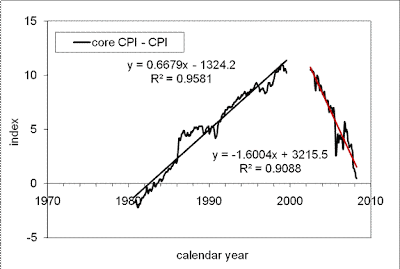Figure 2. Linear regression of the difference between the core CPI and CPI.Figure 3. Extrapolation of the trend in the differnce between core CPI and CPI beyond 2007 .Figure 4. The return to the new linear trend. An overshoot below the trend line may happen in 2009 or early 2010. Then, the differnce will align around the new trend, which will emerge promptly.

References
 Kitov, I., Kitov, O., (2008). Long-Term Linear Trends In Consumer Price Indices, Journal of Applied Economic Sciences, Spiru Haret University, Faculty of Financial Management and Accounting Craiova, vol. III(2(4)_Summ), pp. 101-112. http://www.jaes.reprograph.ro/articles/Kitov.pdf

 Kitov, I., Kitov, O., (2009). A fair price for motor fuel in the United States, MPRA Paper 15039, University Library of Munich, Germany, http://mpra.ub.uni-muenchen.de/15039/01/MPRA_paper_15039.pdf

 Kitov, I., Kitov, O., (2009). Apples and oranges: relative growth rate of consumer price indices, MPRA Paper 13587, University Library of Munich, Germany, http://mpra.ub.uni-muenchen.de/13587/,
http://mpra.ub.uni-muenchen.de/13587/01/MPRA_paper_13587.pdf

 Kitov, I., Kitov, O., (2009). Sustainable trends in producer price indices, MPRA Paper 15194, University Library of Munich, Germany, http://mpra.ub.uni-muenchen.de/15194/01/MPRA_paper_15194.pdf

### Price inflation: Q&A

Sometimes Q&A is the best way to present new concepts. I expect this post to grow over time and welcome questions related to theoretical and empirical aspects of price inflation in developed countries. Emerging and developing countries are also of interest, especially the cases of hyperinflation.

Q1. What is it - price inflation?
In narrow sense, price inflation is a reaction of economic system to changing level of labor force. Inflation is the process which recovers personal income distribution to its natural and fixed (rigid) shape. As a monetary process, inflation is expressed in changing prices for goods and services (G&S), with the total cost of all these G&S exactly determined by real economic growth, if any, and inflation, as predefined by the change in labor force level.
In developed (capitalist) economies, prices for G&S have to interact and compete, i.e. to be governed by "invisible hand", in order to find some final (but not predetermined!) configuration, which corresponds to the total nominal cost of the G&S. This total cost (nominal GDP) is defined by real economic growth and inflation.
(Detailed explanation of price inflation as an economic phenomenon and process as well as relevant quantitative analysis are given in our papers.)

Q2. Is it possible to describe the link between inflation and labor force change using simple mathematical representation?
There exists a linear lagged relationship between inflation, π(t), unemployment, UE(t), and the change rate of labor force, dLF(t)/LF(t):

π(t+t1)= AdLF(t)/LF(t) + BUE(t+t2) + C

where A, B, and C are country-specific empirical constant (this relationship is normalized to inflation); t1 and t2 are time lags between the change in labor force and the reaction of inflation and unemployment. These lags may reach several years and there is no general rule which variable reacts first.

Q3. Is inflation an economic ill, constantly threatening economic stability?
There is no statistical or any quantitative link between inflation and real economic growth except the one, which is related to population changes. There is a formal statistical and econometric prove available in our papers that real economic growth depends only on the attained level of real GDP per capita and the change rate of population of a country-specific age. This defining age is nine years in the USA.

Q4. Is deflation harmful?
Since real economic growth and inflation are not directly related, the recession and deflation (like observed in Japan) have different sources. For example, the former process is approaching its end in Japan and real economic growth (per capita) is on its potential level (http://inflationusa.blogspot.com/2007/07/modelling-evolution-of-real-gdp-in.html). The latter process will last the next 40 years with increasing amplitude (http://inflationusa.blogspot.com/2007/08/prediction-of-cpi-inflation-in-japan.html )

Q5. Is inflation unpredictable?
Inflation can be accurately predicted at various time horizons in developed countries. Examples are the USA, Japan, France, Germany, Canada, Austria, and the UK. The accuracy of inflation prediction is completely defined by the accuracy of labor force measurements, as follows from the relationship in Q2. There are some simple means to improve current labor force estimates.

Q6. Do central bankers control inflation and inflationary expectations through clear inflation targeting?
Because inflation is completely defined by the change rate of labor force Central Banks do not control inflation as it is. They can potentially control the balance between inflation and unemployment, which are linear lagged function of the change rate of labor force.
Quantitative prediction of the history of inflation in USA is a good example that the Fed does not control inflation. The history of inflation in France demonstrates that the suppression of money supply results in elevated unemployment. So, this is an example of negative affect of central bank in sense of high unemployment balancing low inflation.

## 9/16/09

### ConocoPhillips share price revisited

Since September 16, the readings of the headline CPI and its components for August 2009 are available (we retrieve all CPI data from http://www.bls.gov/data). We recalculate our model for selected stock prices from the S&P 500 list. First is ConocoPhillips since it provides a good example of a company, which share price has been leading defining components of the CPI. In the previous posts and articles [1,2] we introduced several models with varying number of CPI components. Here we compare two models. The first one, we call it 3-C model, is seeking those two CPI components from 34 pre-selected ones, which minimize the difference between observed (monthly closing price adjusted for dividends and splits) and predicted prices for the period between July 2003 and August 2009. This model also includes free term (constant) and linear time term [3-6], which compensates well know linear (time) trends between various CPI components. The second model (5-C) uses four CPI components and free term. The number of potential defining components is reduced to ten: food (F), housing (H), apparel (A), transportations (T), medical care (M), recreation (R), education and communication (EC), other goods and services (O), the headline CPI (C), and the core CPI (CC). These components compile a full set of the CPI expenditure categories, and also comprise a subset of the 34-component set.
The 3-C model for COP is as follows:

COP(t)= 3.41CF(t+1) - 7.17EC(t-6) + 7.22*(t-2000) + 145.76 (1)

where CF is the headline CPI less food. It does not differ from the model presented in the previous post except the time shifts are +1 (was +2) month and -6 (was -5) months now. This is due to the inclusion on the CPI readings for August. Figure 1 shows the predicted and observed curves. Standard deviation between the curves is \$3.96 for the period between July 2003 and August 2009.Figure 1. Observed and 3-C predicted COP’s share prices.

The 5-C model does not include the linear time term, but two components extra to the 3-C model play this role. The best 5-C model also shows that the stock price leads all defining CPI components:

COP(t)= 1.53H(t+3) – 8.29R(t+1) – 4.90EC(t-6) + 3.53C(t+1) + 518.4 (2)

There components from the four defining lag behind COP share price and only the EC leads by six months. Figure 2 presents the model. Standard deviation between the curves is only \$3.03 for the period between July 2003 and August 2009, which is better than the accuracy of the 3-C model.Figure 2. Observed and 5-C predicted COP’s share prices.

At first glance, the 5-C prediction is better that the 3-C one. However, this conclusion is not fully correct. As mentioned above, the extra two components chiefly represent the linear trend. Figure 2 illustrates the phenomenon. The difference between R and EC actually provides a linear trend with small fluctuations, which slightly suppress the residual of the 3-C model. Among all pairs of CPI components -8.29R(t+1) – 4.90EC(t-6) suppresses the model residual (noise) the most efficient. It is a positive side of the 5-C model. It has a negative side as well. The difference may diverge from the linear trend in the next several months and will increase the residual and thus the accuracy of prediction instead of suppression. So, we do prefer using the 3-C model for the prediction of share prices. Figure 4 depicts both residuals.Figure 3. Two differences between defining components in (2).

We will continue updating both empirical models. The next obvious date is October 1, 2009 with the next closing share price for September.
References
 Kitov, I., Kitov, O., (2009). Predicting share price of energy companies: June-September 2009, MPRA Paper 15863, University Library of Munich, Germany, http://mpra.ub.uni-muenchen.de/15863/01/MPRA_paper_15863.pdf
 Kitov, I., Kitov, O., (2009). Modelling selected S&P 500 share prices, MPRA Paper 15862, University Library of Munich, Germany, http://mpra.ub.uni-muenchen.de/15862/01/MPRA_paper_15862.pdf
 Kitov, I., Kitov, O., (2008). Long-Term Linear Trends In Consumer Price Indices, Journal of Applied Economic Sciences, Spiru Haret University, Faculty of Financial Management and Accounting Craiova, vol. 3(2(4)_Summ), pp. 101-112.
 Kitov, I., (2009). Apples and oranges: relative growth rate of consumer price indices, MPRA Paper 13587, University Library of Munich, Germany, http://mpra.ub.uni-muenchen.de/13587/01/MPRA_paper_13587.pdf
 Kitov, I., Kitov, O., (2009). A fair price for motor fuel in the United States, MPRA Paper 15039, University Library of Munich, Germany, http://mpra.ub.uni-muenchen.de/15039/01/MPRA_paper_15039.pdf
 Kitov, I., Kitov, O., (2009). Sustainable trends in producer price indices, MPRA Paper 15194, University Library of Munich, Germany, http://mpra.ub.uni-muenchen.de/15194/01/MPRA_paper_15194.pdf